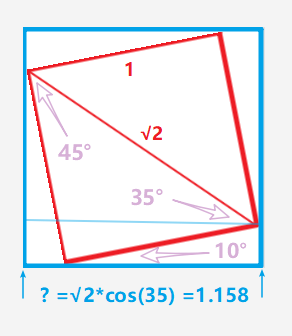# Lesson 3: Couldn't understand data.resize() for multi label classification for planet data set?

When we were doing multilabel classification om planet dataset, we decreased the size of the data from 256 to 64. After augmenting data we have done,

data = data.resize(int(sz*1.3), ‘tmp’)

Jeremy says it’s for computational advantage resulting into speed-up. But don’t really understand, how exactly we would speed up? Do we ignore all the images with size greater than or equal to sz*1.3 and hence speed up, but that would be lots of images we would be not looking onto and will lead to limited learning.

1 Like

the `data.resize()` is mentioned in Lesson 2, and I also don’t understand what it’s doing.

Haven’t been able to understand what it’s doing either.

Is resizing the images and copy their resized version in ‘tmp’ folder. I don’t see why he multiplies the size by 1.3.
Maybe the speed up is due to the fact that he saves the images in tmp folder.

``````def resize_imgs(fnames, targ, path, new_path):
if not os.path.exists(os.path.join(path,new_path,str(targ),fnames)):
ims = e.map(lambda x: resize_img(x, targ, path, 'tmp'), fnames)
for x in tqdm(ims, total=len(fnames), leave=False): pass
return os.path.join(path,new_path,str(targ))``````

Reading notes by other classmates plus my own understanding, here is what I think.

When we specify what transforms to apply, we pass a size `sz` to `tfms_from_model`:

``````tfms = tfms_from_model(f_model, sz, aug_tfms=transforms_top_down,
max_zoom=1.05)
``````

After the assignment, `tfms` will pass to the data loader `ImageClassifierData.from_csv(...)`. One of the things the data loader does is to resize the images on the fly. In other words, the images go into the data loader in full size, and it ONLY resizes the image when it outputs them to the model via Python’s generator.

Notice calling `data.resize(int(sz * 1.3), 'tmp')` allow us to resize the images in advanced. Here, `data.resize()` takes a maximum size (e.g. `sz=64`, so the maximum size is `int(sz * 1.3)` = `83`), so each image has dimension bigger than `sz * 1.3` will be resized. Since images can be rectangular, `data.resize()` will make sure the smallest dimension to be `sz * 1.3` and center-crop it.

Importantly, it creates a resized copy of the entire dataset to a temporary folder `tmp`.

This technique will save you a lot of time because according to Jeremy, if you have large images, say 1000 by 1000, reading the images and resizing it to 64 by 64 before doing SGD during training will take more time than training the convolution nets alone! By calling `data.resize()` in advanced, we can resize the images once and do SGD multiple times efficiently.

16 Likes

I was talking about data.resize(), this is resize_imgs. Does this function called eventually?

Thanks. I understand this better now. Does it resize to sz or sz*1.3?

These files are saved in the ./tmp/ folder. You can look at there and see what they look like and what the new dimensions are.

AFAIK, if the dimensions of the image are both smaller than `sz * 1.3`, the image size remains the same. Otherwise, if one of the dimensions is larger than `sz * 1.3`, the smallest dimension will become `sz * 1.3`.

For example, if we have an 100 by 200 image and `sz=64`, using the `1.3` threshold, we want the maximum image dimension to be `sz * 1.3 = 83`. Now since the smallest dimension of the image is 100, we resize the dimension 100 to 83. Next, we center-crop the resized 83 by 200 image, so we’ll end up with a 83 by 83 image.

3 Likes

Why is multiplied by 1.3? why is not simply sz 64?

`data.resize` calls at the very end `resize_imgs`

I’m not sure but I kind of agree with @giusvit that “As for the coefficient, I think it’s try and test to find a balance between quality and speed.” where I think “coefficient” = 1.3.

2 Likes

I believe you should have slightly bigger size image so that data augmentation could work out and generate variation in the actual output images

1 Like

@alessa

The 1.3 came from the randomRotae(10), randomflip(1.05), max_zoom(1.05).

assume the image size is 11.
When we rotate, and crop 1
1 size image. We need crop from a bigger size image.
How big is the size?then we have to do 5% for both flip and zoom.
so the final size will be √2 x cos(35) x 1.05 x 1.05=1.277

6 Likes

Does this work on ImageClassifierData.from_paths? Tried this and I’m getting the error listed below. Been trying for an hour to figure out why this happens, but it beats me…

``````def get_data(sz, bs): # sz: image size, bs: batch size
tfms = tfms_from_model(arch, sz, aug_tfms=transforms_side_on, max_zoom=1.1)
data = ImageClassifierData.from_paths(PATH, 'train', test_name='test', tfms=tfms)
return data if sz > 300 else data.resize(340, 'tmp')

---------------------------------------------------------------------------
TypeError                                 Traceback (most recent call last)
<ipython-input-250-5585e975ed05> in <module>
----> 1 data = get_data(bs, sz)

<ipython-input-248-260ace9fefde> in get_data(sz, bs)
2     tfms = tfms_from_model(arch, sz, aug_tfms=transforms_side_on, max_zoom=1.1)
3     data = ImageClassifierData.from_paths(PATH, 'train', test_name='test', tfms=tfms)
----> 4     return data if sz > 300 else data.resize(340, 'tmp')
5
6 #Source:

C:\ProgramData\Anaconda3\envs\fastai\lib\site-packages\fastai\dataset.py in resize(self, targ_sz, new_path, resume, fn)
440         new_ds = []
441         dls = [self.trn_dl,self.val_dl,self.fix_dl,self.aug_dl]
--> 442         if self.test_dl: dls += [self.test_dl, self.test_aug_dl]
443         else: dls += [None,None]
444         t = tqdm_notebook(dls)